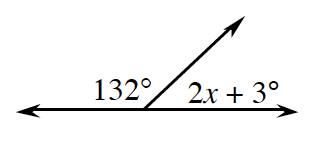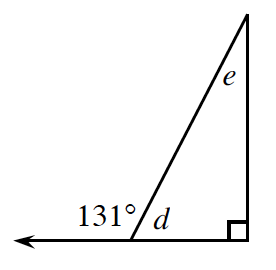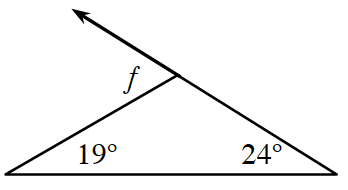### Home > CCAA > Chapter 9 Unit 10 > Lesson CC3: 9.1.3 > Problem9-34

9-34.

In each angle problem below, solve for the variable(s). Write the names of the definition(s) and relationship(s) that justify the steps in your solution.

1.$132 + (2x + 3) = 180$

1.The angle measuring $131º$ is an exterior angle to the triangle.

$d + 131 = 180$

$d = 49º$

$d + e + 90 = 180$
or
$131 = e + 90$

$e = 41º$

1.An exterior angle is equal to the sum of the remote interior angles.